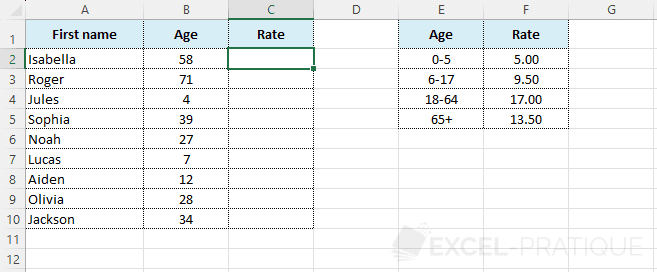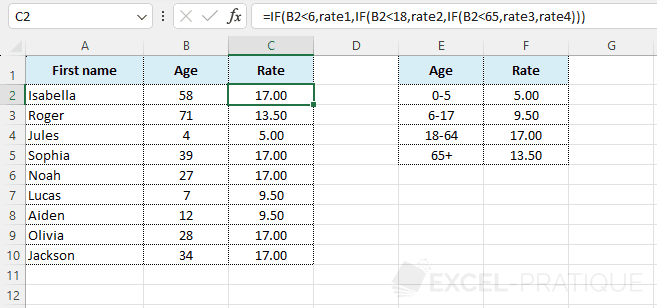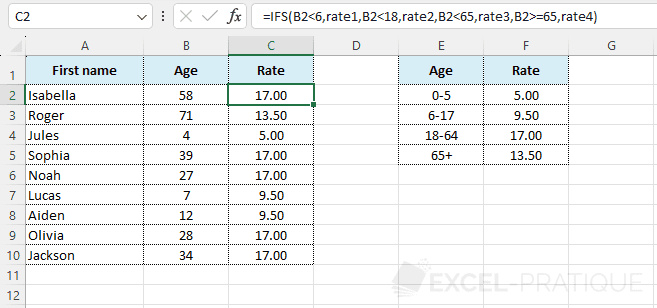# Excel Function: IFS

The IFS function checks whether one or more conditions are met and returns a value corresponding to the first true condition.

Prerequisite: understand the IF function

Usage:

`=IFS(logical_test1, value_if_true1)`

or

`=IFS(logical_test1, value_if_true1, logical_test2, value_if_true2, ...)`

## Usage Example

The goal here will be to display the rate based on each person's age:For better readability of formulas, the rate cells have been named from "rate1" to "rate4".

Before the appearance of the IFS function, it was necessary to nest IF functions for each additional criteria, for example:

``=IF(B2<6,rate1,IF(B2<18,rate2,IF(B2<65,rate3,rate4)))``The IFS function avoids all these nesting and simply adds as many pairs (logical test -> value if true) as necessary:

``=IFS(B2<6,rate1,B2<18,rate2,B2<65,rate3,B2>=65,rate4)``